Ex 9.4

Chapter 9 Class 12 Differential Equations
Serial order wise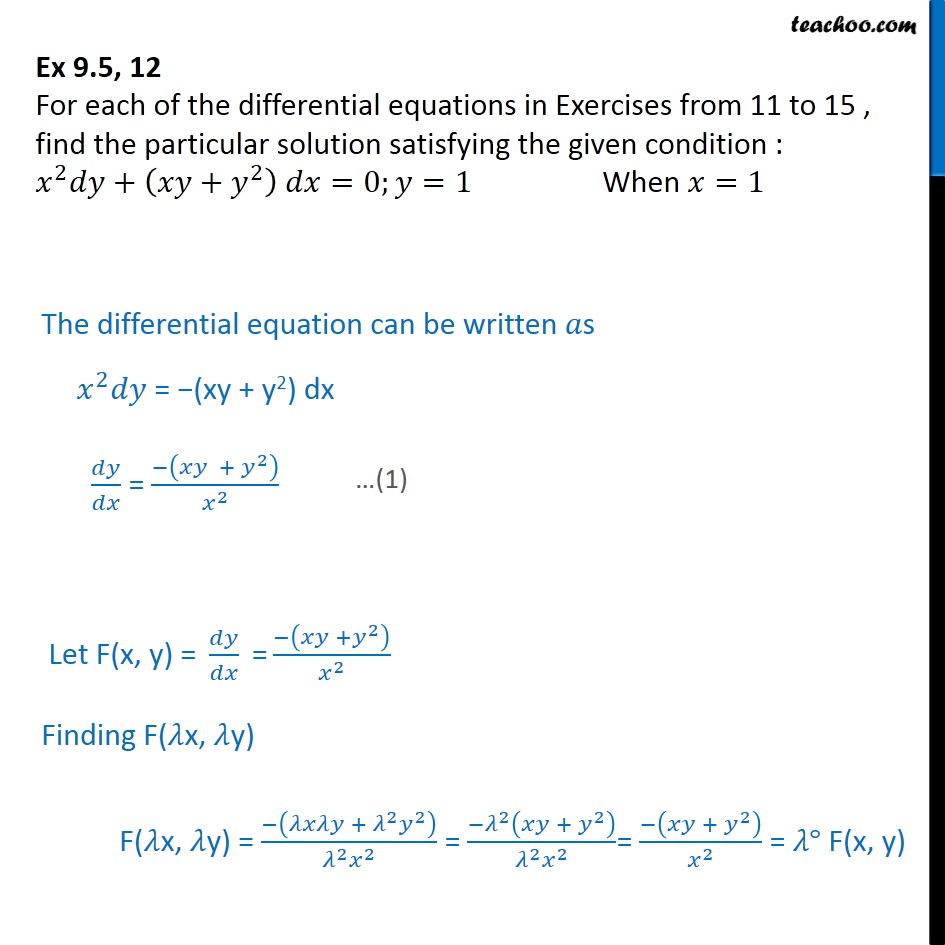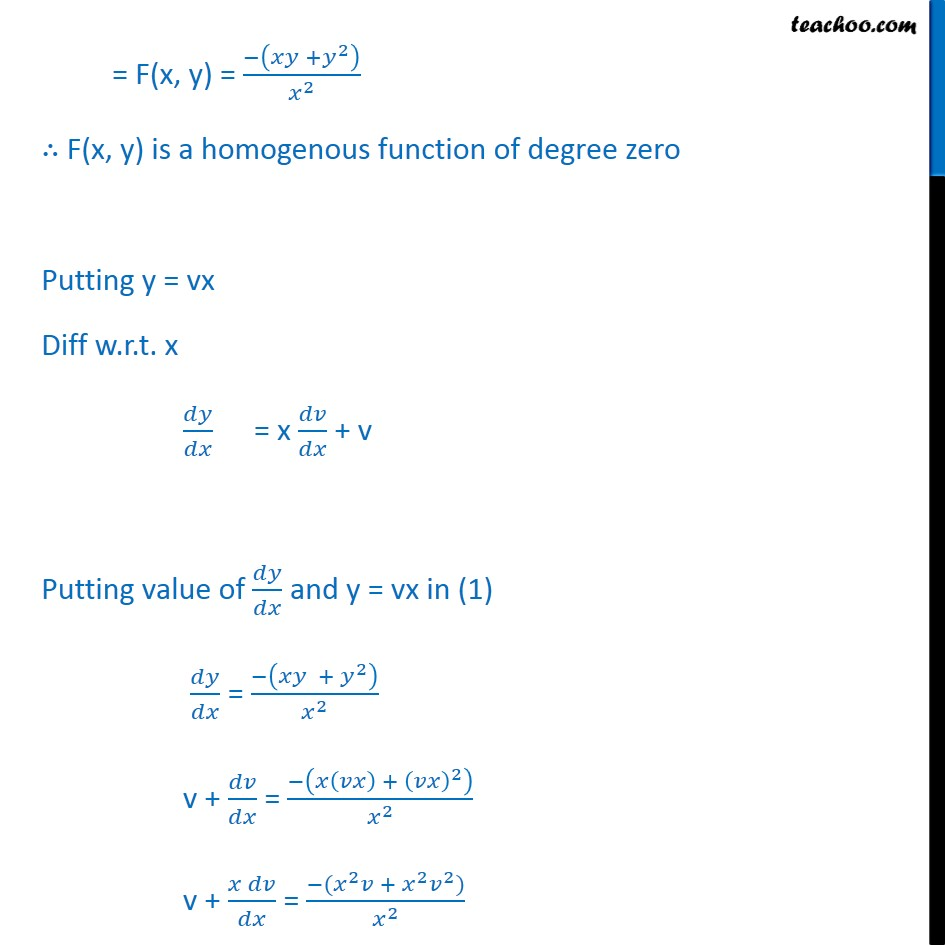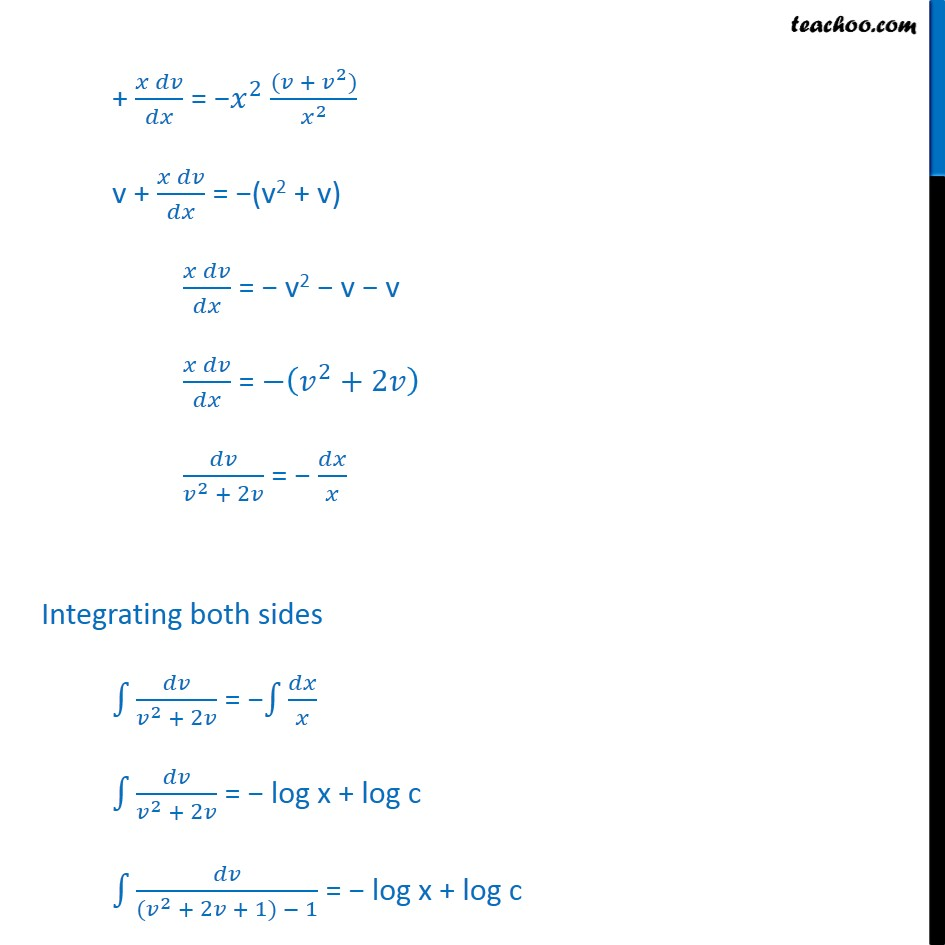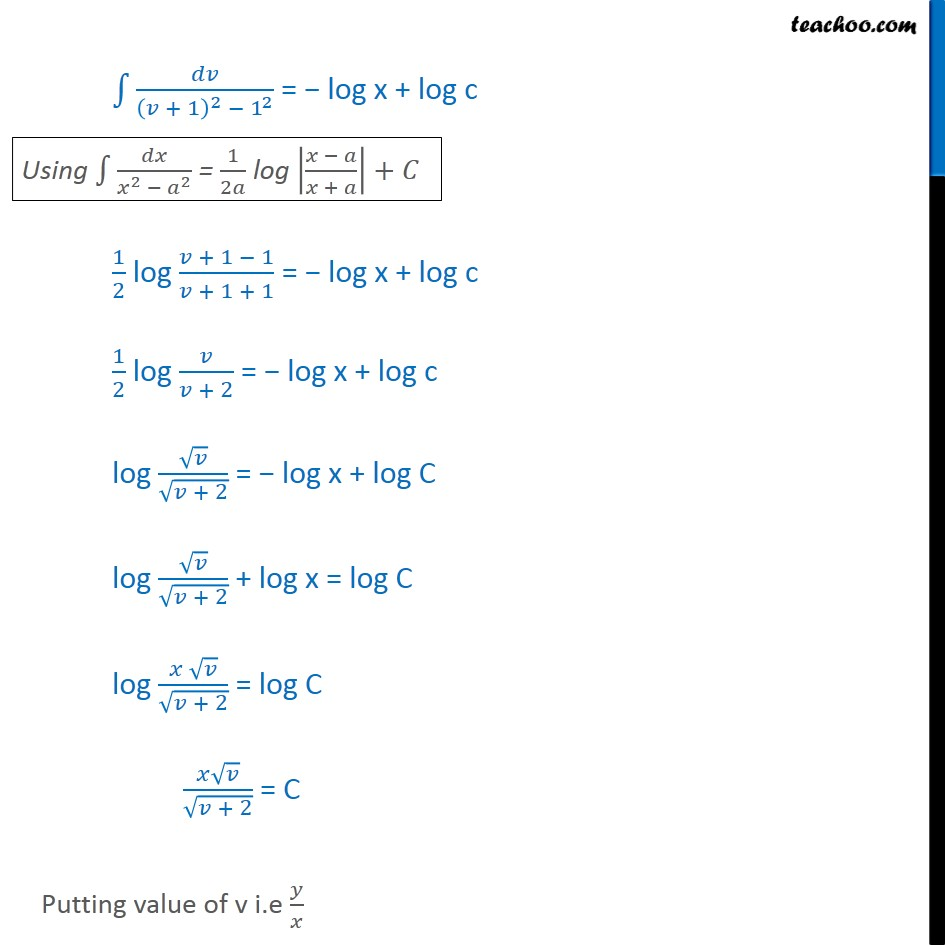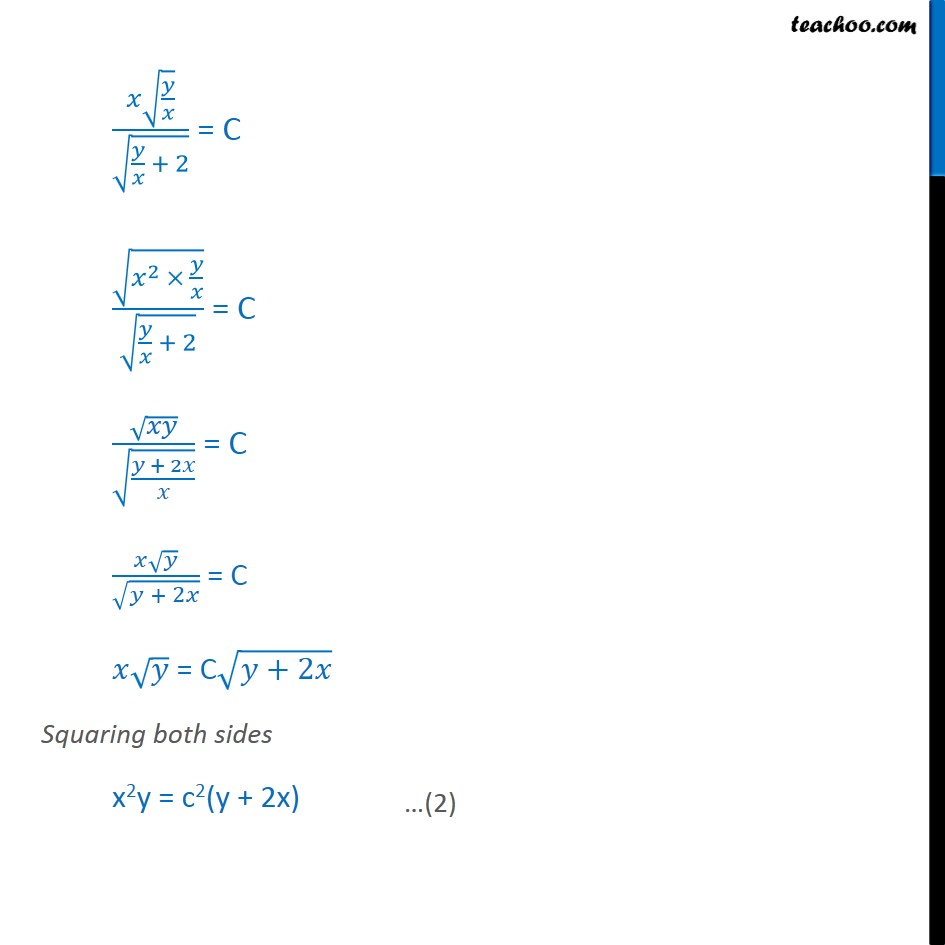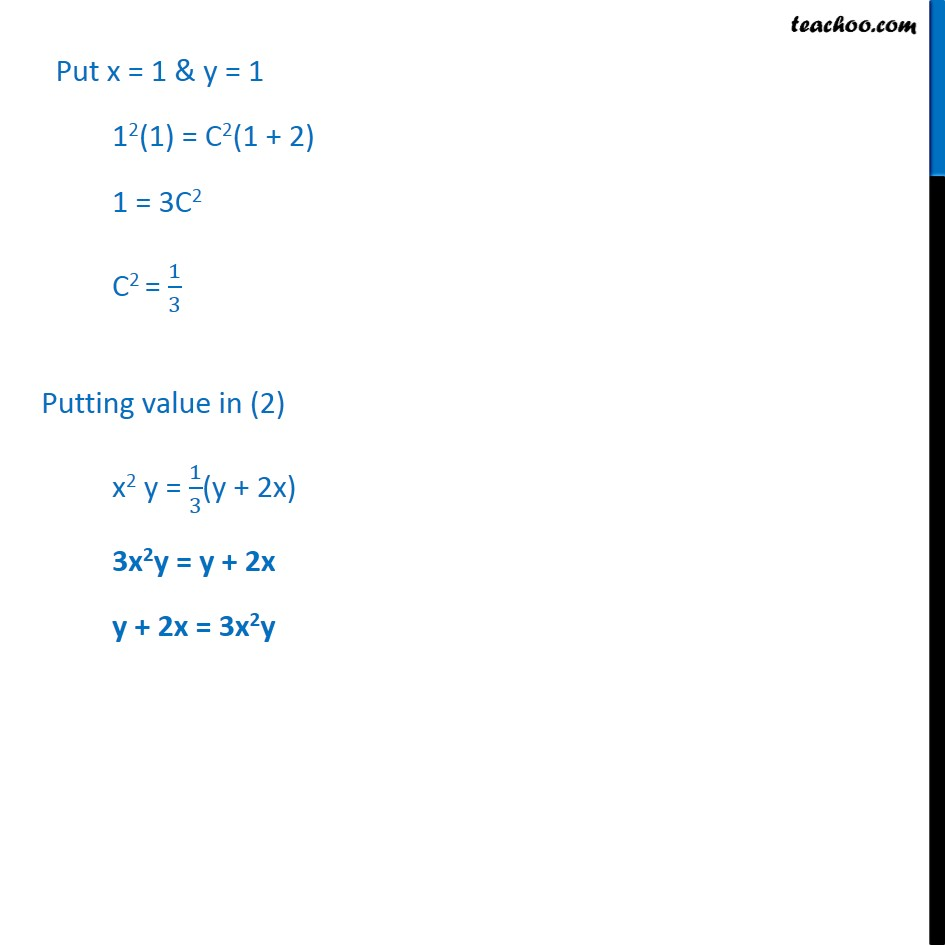Learn in your speed, with individual attention - Teachoo Maths 1-on-1 Class

### Transcript

Ex 9.4, 12 For each of the differential equations in Exercises from 11 to 15 , find the particular solution satisfying the given condition : 𝑥﷮2﷯𝑑𝑦+ 𝑥𝑦+ 𝑦﷮2﷯﷯ 𝑑𝑥=0;𝑦=1 When 𝑥=1 The differential equation can be written 𝑎s 𝑥﷮2﷯𝑑𝑦 = −(xy + y2) dx 𝑑𝑦﷮𝑑𝑥﷯ = − 𝑥𝑦 + 𝑦﷮2﷯﷯﷮ 𝑥﷮2﷯﷯ Let F(x, y) = 𝑑𝑦﷮𝑑𝑥﷯ = − 𝑥𝑦 + 𝑦﷮2﷯﷯﷮ 𝑥﷮2﷯﷯ Finding F(𝜆x, 𝜆y) F(𝜆x, 𝜆y) = − 𝜆𝑥𝜆𝑦 + 𝜆﷮2﷯ 𝑦﷮2﷯﷯﷮ 𝜆﷮2﷯𝑥﷮2﷯﷯ = − 𝜆﷮2﷯ 𝑥𝑦 + 𝑦﷮2﷯﷯﷮ 𝜆﷮2﷯𝑥﷮2﷯﷯= − 𝑥𝑦 + 𝑦﷮2﷯﷯﷮ 𝑥﷮2﷯﷯ = 𝜆° F(x, y) = F(x, y) = − 𝑥𝑦 + 𝑦﷮2﷯﷯﷮ 𝑥﷮2﷯﷯ ∴ F(x, y) is a homogenous function of degree zero Putting y = vx Diff w.r.t. x 𝑑𝑦﷮𝑑𝑥﷯ = x 𝑑𝑣﷮𝑑𝑥﷯ + v Putting value of 𝑑𝑦﷮𝑑𝑥﷯ and y = vx in (1) 𝑑𝑦﷮𝑑𝑥﷯ = − 𝑥𝑦 + 𝑦﷮2﷯﷯﷮ 𝑥﷮2﷯﷯ v + 𝑑𝑣﷮𝑑𝑥﷯ = − 𝑥 𝑣𝑥﷯ + 𝑣𝑥﷯﷮2﷯﷯﷮ 𝑥﷮2﷯﷯ v + 𝑥 𝑑𝑣﷮𝑑𝑥﷯ = −( 𝑥﷮2﷯𝑣 + 𝑥﷮2﷯ 𝑣﷮2﷯)﷮ 𝑥﷮2﷯﷯ + 𝑥 𝑑𝑣﷮𝑑𝑥﷯ = − 𝑥﷮2﷯ (𝑣 + 𝑣﷮2﷯)﷮ 𝑥﷮2﷯﷯ v + 𝑥 𝑑𝑣﷮𝑑𝑥﷯ = −(v2 + v) 𝑥 𝑑𝑣﷮𝑑𝑥﷯ = − v2 − v − v 𝑥 𝑑𝑣﷮𝑑𝑥﷯ = − 𝑣﷮2﷯+2𝑣﷯ 𝑑𝑣﷮ 𝑣﷮2﷯ + 2𝑣﷯ = − 𝑑𝑥﷮𝑥﷯ Integrating both sides ﷮﷮ 𝑑𝑣﷮ 𝑣﷮2﷯ + 2𝑣﷯﷯ = − ﷮﷮ 𝑑𝑥﷮𝑥﷯﷯ ﷮﷮ 𝑑𝑣﷮ 𝑣﷮2﷯ + 2𝑣﷯﷯ = − log x + log c ﷮﷮ 𝑑𝑣﷮ (𝑣﷮2﷯ + 2𝑣 + 1) − 1﷯﷯ = − log x + log c ﷮﷮ 𝑑𝑣﷮ 𝑣 + 1﷯﷮2﷯ − 1﷮2﷯﷯﷯ = − log x + log c 1﷮2﷯ log 𝑣 + 1 − 1﷮𝑣 + 1 + 1﷯ = − log x + log c 1﷮2﷯ log 𝑣﷮𝑣 + 2﷯ = − log x + log c log ﷮𝑣﷯﷮ ﷮𝑣 + 2﷯﷯ = − log x + log C log ﷮𝑣﷯﷮ ﷮𝑣 + 2﷯﷯ + log x = log C log 𝑥 ﷮𝑣﷯﷮ ﷮𝑣 + 2﷯﷯ = log C 𝑥 ﷮𝑣﷯﷮ ﷮𝑣 + 2﷯﷯ = C Putting value of v i.e 𝑦﷮𝑥﷯ 𝑥 ﷮ 𝑦﷮𝑥﷯﷯﷮ ﷮ 𝑦﷮𝑥﷯ + 2﷯﷯ = C ﷮ 𝑥﷮2﷯ × 𝑦﷮𝑥﷯﷯﷮ ﷮ 𝑦﷮𝑥﷯ + 2﷯﷯ = C ﷮𝑥𝑦﷯﷮ ﷮ 𝑦 + 2𝑥﷮𝑥﷯﷯﷯ = C 𝑥 ﷮𝑦﷯﷮ ﷮𝑦 + 2𝑥﷯﷯ = C 𝑥 ﷮𝑦﷯ = C ﷮𝑦+2𝑥﷯ Squaring both sides x2y = c2(y + 2x) Put x = 1 & y = 1 12(1) = C2(1 + 2) 1 = 3C2 C2 = 1﷮3﷯ Putting value in (2) x2 y = 1﷮3﷯(y + 2x) 3x2y = y + 2x y + 2x = 3x2y